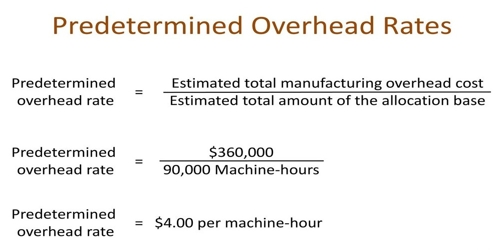# Pre-determined Overhead Rate

Pre-determined Overhead Rate

A predetermined rate is a probable amount of overhead costs that managerial accountants calculate an activity base will use. Predetermined overhead rate is used to apply manufacturing overhead to products or job orders and is usually computed at the beginning of each period, by dividing the, estimated manufacturing overhead cost by an allocation base (also known as activity base or activity driver). It is an allocation rate that is used to apply the estimated cost of manufacturing overhead to cost objects for a specific reporting period. Commonly used allocation bases are direct labor hours, direct labor dollars; machine hours, and direct materials.

Formula: This formula refers to the predetermined overhead because this overhead total is based on estimations, rather than the actual cost. The formula of the predetermined overhead rate is written as follows:

Predetermined overhead rate = [Estimated manufacturing overhead cost / Estimated total units in the allocation base].

A number of possible allocation bases are available for the denominator, such as direct labor hours, direct labor dollars, and machine hours.

Pre-determined Overhead Rate is used to recognize the probable costs of production, which allows the business to appropriately distribute the financial resources needed to make sure suitable and resourceful production and operations. A company uses this rate to allocate overhead costs to the costs of products.